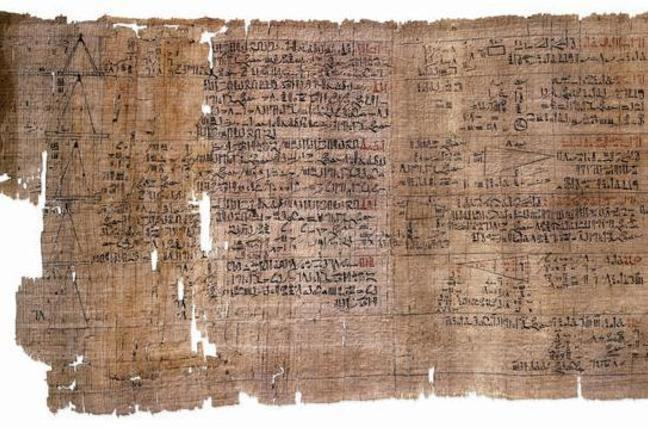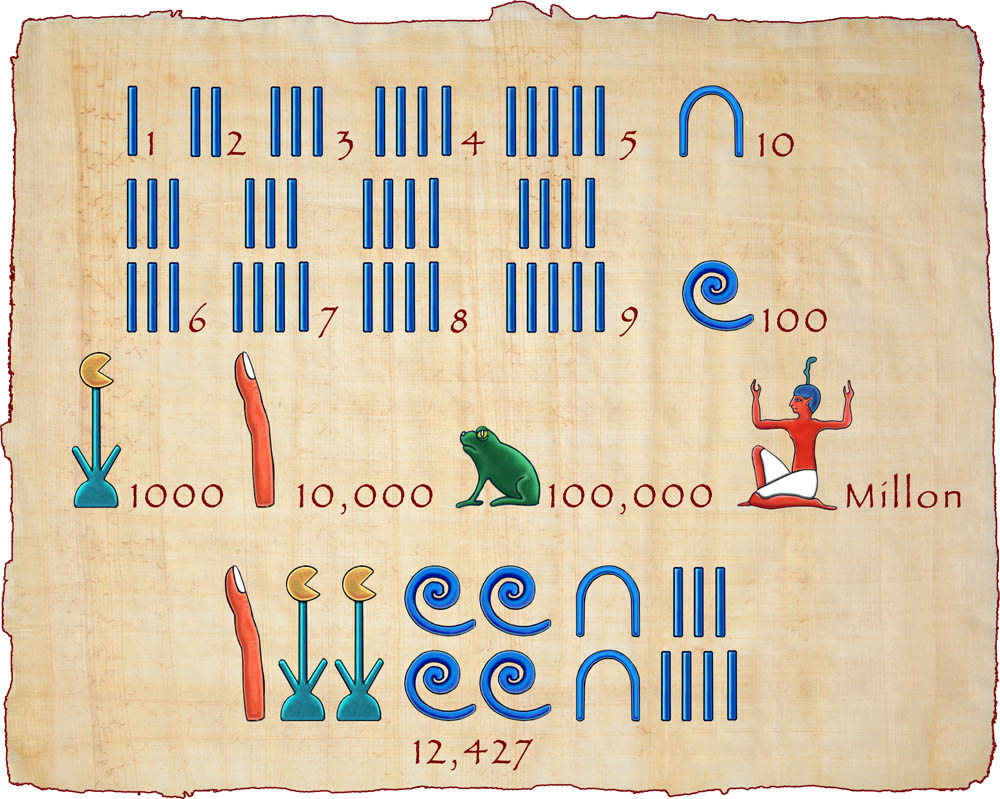## Want to keep learning?

This content is taken from the Davidson Institute of Science Education at the Weizmann Institute of Science's online course, Maths Puzzles: Cryptarithms, Symbologies and Secret Codes. Join the course to learn more.
2.2

## Davidson Institute of Science Education at the Weizmann Institute of ScienceThe Rhind Papyrus

# Ancient Egyptian Symbologies

## Introduction

The term symbology is used to describe a system in which different symbols or signs are used to represent information be it letters, numbers, words or instructions. Mankind has always used different symbologies. There are many examples: Roman numerals, Egyptian hieroglyphs, Braille, Morse code, and in the modern world – barcodes and different computer codes. There is a lot of resemblance between symbology and cryptography (secret writing), but there is also one main difference. In cryptography, the goal is to hide information from people that are not authorized. In symbology, the goal is to represent the information in an efficient and convenient manner.

## Numbers in the ancient world

People have always needed to count things. Before numbers were written the way they are today, other methods were used. The simplest method was to use rods or draw lines to represent different amounts. For example, if a merchant in the market was faced with two groups of wine barrels, one group with three barrels, and the other group with six, he had to calculate the total sum and it would have been written using pictures of rods.

With time, different cultures developed different symbols to represent numbers. As early as 5000 years ago, the ancient Egyptians carved in stone their pictorial symbology, hieroglyphs, to communicate ideas and to write words and numbers. Each drawing or symbol had several interpretations depending on the context. One meaning could be simply the object depicted in the drawing. The drawing gets a completely different meaning altogether when combined with another one. In that case, the combination of the two symbols would express the meaning. Let’s demonstrate this principle in English. A drawing of a star might mean: star, but the shape of a star together with a rock, could mean ‘rock star’, as in ‘Lady Gaga’. Hieroglyphs were mostly carved in stone.

There were pictures for numbers. A rod for the number one, an ox strap for the number ten, a coil of rope for one hundred, a lotus plant for a thousand and so on. Here is a chart of Hieroglyphic numbers:Although positional notation was already in use thousands of years ago by the Babylonians (except for the use of zero), it was not used by the Egyptians. So, the hieroglyphic number 2 was the drawing of two rods, and the hieroglyphic number 5072 was depicted by engraving 5 lotus plant, seven ox straps and two rods. In principle, there is no need to write the symbols in any specific order, since the position had no meaning, but hieroglyphic numbers were usually written with symbols of larger numbers to the left, or on top of the smaller ones. Here is the Egyptian symbol for the number: 4622, one of the numbers carved on the walls of the temple in Karnak (Thibes), Egypt.The Egyptians also used a more popular writing symbology, hieratic script, which was kind of a shorthand of hieroglyphics. The famous Rhind papyrus, depicted in the image above and kept in the British Museum in London, from which we know a lot about Egyptian mathematics, was written in Hieratic script. It is named after the Scottish archeologist, Alexander Henry Rhind, who found it, and was written in ink on papyrus (like nearly all hieratic scripts) by an Egyptian scribe called Ahmes. The Rhind papyrus tells us a lot about how the Egyptians performed many of their mathematical calculations. It includes every day calculation problems of many kinds: addition, subtraction, multiplication and division. It also reveals a basic awareness of some complicated math, such as composite and prime numbers, several types of means, and perfect numbers. One of the most intriguing features of ancient Egyptian math is the way they treated fractions.

## Egyptian fractions

One interesting hieroglyph used by the Egyptians in the context of numbers is a picture of an eye. An “eye” above a number indicates what part it is from the whole number. An eye above the number 3, for example, indicates that this is the third part of the whole number, i.e. the fraction one third (1/3). So, this hieroglyph turns the number into its inverse. One might think that the “eye” simply represents fractions, but there is a significant difference between Egyptian fractions and the fractions that we use today. While nowadays we write fractions like 2/5 or 3/5, where the numerator can be any number, in Egyptian fractions the numerator is always 1: ½, ¼, etc. These fractions are known as “unit fractions”. But if the Egyptians only used unit fractions, how did they write fractions like 3/4 or 7/12? These fractions were written as “sums” of fractions, each one different from the other. For example, the fraction 3/4 can be written as 1/2+1/4. If we were asked today what is 1/3 + 1/4? - we would write: 7/12, but the Egyptians would just leave it as 1/3+1/4. One notes in passing that the Egyptians had one exception to the rule - the fraction 2/3.

Why did the Egyptians use only ‘unit fractions’? There have been a few speculations, but here is one which seems to me a good guess. Look at the following problem for example: In a pizzeria, the vendor has to divide 5 pizza trays between 8 people equally, how can he do this?

• In today’s mathematical language we could say that each of them has to get 5/8 of the pizzas. The vendor would have to divide each tray into eight portions so that everyone gets one piece from every tray. This would create a lot of work for the vendor. Alternatively, he could give one person 5/8 of one pizza, the second the remaining 3/8 and another 2/8 from the second pizza, the third person would get 5/8 etc.
• Another option is to write 5/8 as an Egyptian fraction: 1/2+1/8 = 5/8. In this case, the vendor could take 4 pizzas and cut each one of them into half so everyone gets half a pizza, while only the last pizza he would have to cut into eight slices so that everyone gets an additional eighth.

Not only does the Egyptian method result in a small number of slices for each person, it is also perceived by them to be fairer. Everyone will get two pieces of pizza, one the size of a half and the other the size of an eighth, instead of some getting one piece (5/8) and others two (3/8 + 2/8).

Mathematicians are still intrigued by Egyptian fractions today, and there is much still to be discovered.

## Interested in learning more about Egyptian symbologies?

Click here for a brief introduction to Egyptian scripts - and then mark this step as complete and press next to see how well you can decipher Egyptian numerals.